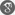##### Cite as:
Abimbola Abolarinwa, A New Entropy Formula and Gradient Estimates for the Linear Heat Equation on Static Manifold, Int. J. Anal. Appl., 6 (1) (2014), 1-17.

#### Abstract

In this paper we prove a new monotonicity formula for the heat equation via a generalized family of entropy functionals. This family of entropy formulas generalizes both Perelman’s entropy for evolving metric and Ni’s entropy on static manifold. We show that this entropy satisfies a pointwise differential inequality for heat kernel. The consequences of which are various gradient and Harnack estimates for all positive solutions to the heat equation on compact manifold.

#### References

1. A. Abolarinwa, Differential Harnack and logarithmic Sobolev inequalities along RicciHarmonic map flow, To appear2. A. Abolarinwa, Analysis of eigenvalues and conjugate heat kernel under the Ricci flow, PhD Thesis, University of Sussex, (2014).3. D. Bakry, D. Concordet and M. Ledoux, Optimal heat kernel bounds under logarithmic Sobolev inequalities, ESAIM Probab. Statist., 1,(1995), 391-407.4. D. Bakry and M. Ledoux, A logarithmic Sobolev form of the Li-Yau parabolic inequality, Revist. Mat. Iberoamericana 22, (2006), 683-702.5. J. Cheeger and S-T. Yau, A lower bound for the heat kernel, Comm. Pure Appl. Math. 34(4)(1981), 465-480.6. B. Chow, S. Chu, D. Glickenstein, C. Guenther, J. Idenberd. T. Ivey, D. Knopf, P. Lu, F. Luo and L. Ni, The Ricci Flow: Techniques and Applications. Part II, Analytic Aspect, AMS, Providence, RI, (2008).7. E. B. Davies, Heat Kernel and Spectral theory. Cambridge University Press (1989).8. N. Garofalo and E. Lanconelli, Asymptotic behaviour of fundamental solutions and potential theory of parabolic operators with variable coefficients, Math. Ann. 283(2)(1989), 211-239.9. L. Gross Logarithmic Sobolev inequalities, America J. Math 97(1)(1975), 1061-1083.10. L. Gross Logarithmic Sobolev inequalities and contractivity properties of semigroups, Dirichlet Form, Lecture Notes in Mathematics Volume 1563, (1993), 54-88.11. R. Hamilton, A matrix Harnack estimate for the heat equation, Commun. Anal. Geom., 1, (1993), 113-126.12. G. Huang, Z. Huang, H. Li, Gradient estimates and differential Harnack inequalities for a nonlinear parabolic equation on Riemannian manifolds, Ann. Glob. Anal. Geom., 23(3) (1993), 209-232.13. S. Kuang, Qi S. Zhang, A gradient estimate for all positive solutions of the conjugate heat equation under Ricci flow, J. Funct. Anal., 255(4)( 2008), 1008-1023.14. J. Li, X. Xu, Differential Harnack inequalities on Riemannian manifolds I: Linear heat equation, Advances in Math., 226 (2011), 4456-4491.15. P. Li, S-T. Yau, On the parabolic kernel of the Schr¨odinger operator, Acta Math. 156 (1986), 153-20116. R. M¨uller, Differential Harnack Inequalities and the Ricci Flow. European Mathematics Society, (2006).17. L. Ni, The Entropy Formula for Linear Heat Equation, Journal of Geom. Analysis 14(2)(2004), 86-9618. L. Ni, Addenda to ”The Entropy Formula for Linear Heat Equation”, Journal of Geom. Analysis 14(2)(2004), 229-334.19. L. Ni, A note on Perelman’s Li-Yau-Hamilton inequality, Comm. Anal. Geom 14(2006), 883-905.20. G. Perelman, The entropy formula for the Ricci flow and its geometric application, arXiv:math.DG/0211159v1 (2002).21. P. Souplet, Qi S. Zhang, Sharp gradient estimate and Yaus Liouville theorem for the heat equation on noncompact manifolds, Bull. London Math. Soc. 38(2006), 1045-1053.22. F. B. Weissler, Logarithmic Sobolev Inequalities for the Heat-Diffusion Semigroup, Trans Am Math. Soc., 237(1978), 255-269.COPYRIGHT INFORMATIONCopyright © 2020 IJAA, unless otherwise stated.